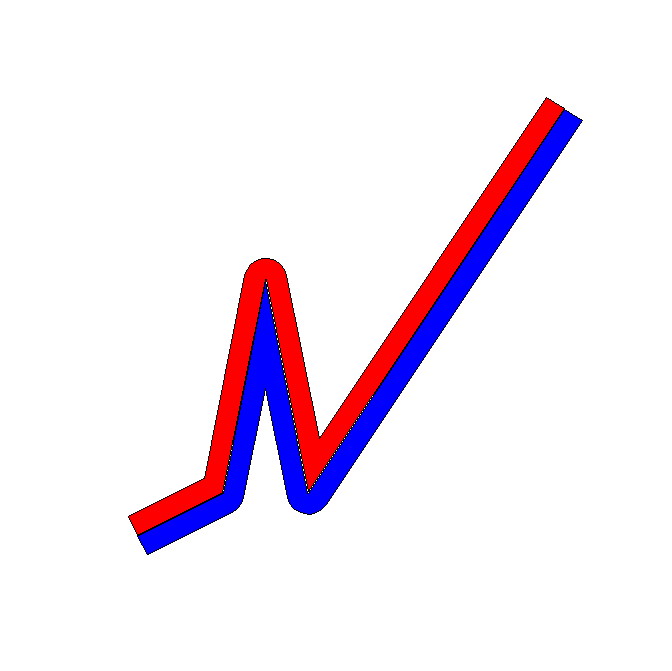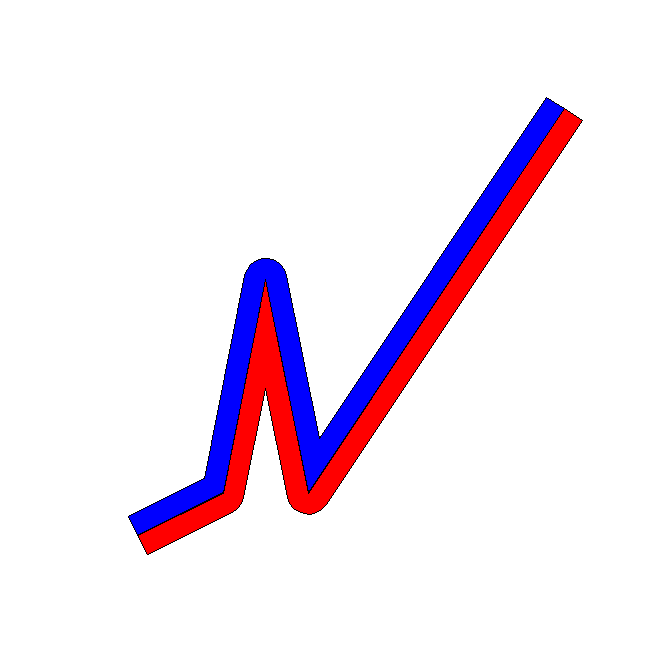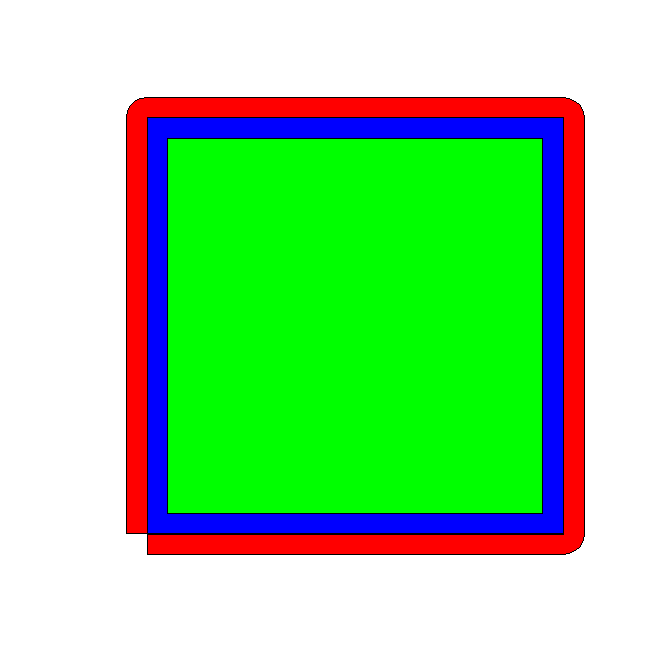# One sided line buffers in R using rgeos

I’ve started to do more geographic data manipulation in R, and part of the reason I do blog posts is for self-reference, so I figured I would share some of the geographic functions I have been working on.

The other day on StackOverflow there was a question that asked how to do one sided buffers in R. The question was closed (and the linked duplicate is closed as well), so I post my response here.

The workflow I describe to make one sided buffers is in a nutshell

• expand the original polyline into a very small area by using a normal buffer, calls this Buf0
• do a normal two sided buffer on the original polyline, without square or rounded ends, call this Buf1
• take the geographic difference between Buf1 and Buf0, which basically splits the original buffer into two parts, and then return them as two separate spatial objects

Here is a simple example.

``````library(rgeos)
library(sp)

TwoBuf <- function(line,width,minEx){
Buf0 <- gBuffer(line,width=minEx,capStyle="SQUARE")
Buf1 <- gBuffer(line,width=width,capStyle="FLAT")
return(disaggregate(gDifference(Buf1,Buf0)))
}

Squig <- readWKT("LINESTRING(0 0, 0.2 0.1, 0.3 0.6, 0.4 0.1, 1 1)") #Orig
TortBuf <- TwoBuf(line=Squig,width=0.05,minEx=0.0001)
plot(TortBuf, col=c('red','blue'))  #First object on left, second on right``````If you imagine travelling along the polyline, which in this example goes from left to right, this is how I know the first red polygon is the left hand and the blue is the right hand side buffer. (To pick a specific one, you can subset like `TortBuf` or `TortBuf`.)

If we reverse the line string, the order will subsequently be reversed.

``````SquigR <- readWKT("LINESTRING(1 1, 0.4 0.1, 0.3 0.6, 0.2 0.1, 0 0)") #Reversed
TortBuf <- TwoBuf(line=SquigR,width=0.05,minEx=0.0001)
plot(TortBuf, col=c('red','blue'))  #Again first object on left, second on right``````Examples of south to north and north to south work the same as well.

``````SquigN <- readWKT("LINESTRING(0 0, 0 1)") #South to North
TortBuf <- TwoBuf(line=SquigN,width=0.05,minEx=0.0001)
plot(TortBuf, col=c('red','blue'))  #Again first object on left, second on right

SquigS <- readWKT("LINESTRING(0 1, 0 0)") #North to South
TortBuf <- TwoBuf(line=SquigS,width=0.05,minEx=0.0001)
plot(TortBuf, col=c('red','blue'))  #Again first object on left, second on right``````

One example in which this procedure does not work is if the polyline creates other polygons.

``````Square <- readWKT("LINESTRING(0 0, 1 0, 1 1, 0 1, 0 0)") #Square
TortBuf <- TwoBuf(line=Square,width=0.05,minEx=0.0001)
plot(TortBuf, col=c('red','blue','green'))

#Switch the direction
SquareR <- readWKT("LINESTRING(0 0, 0 1, 1 1, 1 0, 0 0)") #Square Reversed
TortBuf <- TwoBuf(line=SquareR,width=0.05,minEx=0.0001)
plot(TortBuf, col=c('red','blue','green'))                #Still the same order``````This messes up the order as well. If you know that your polyline is actually a polygon you can do a positive and negative buffer to get the desired effect of interest. If I have a need to expand this to multipart polylines I will post an update, but I have some other buffer functions I may share in the mean time.

### 1 Comment

1.#### Kristina

/  January 18, 2018

Thanks for writing on this topic! On the topic of buffers, do you know how to create a buffer with a variable width? I have a road shapefile with a width attribute that I’d like to base my road buffer off of. For example, if I have a 5 m wide section of road, I’d like my buffer to be 2.5 m on each side. If I have a 10 m wide section of road, I’d like my buffer to be 5 m on each side, etc. I’m not sure if gBuffer has this functionality, but I’d like to do something like this: